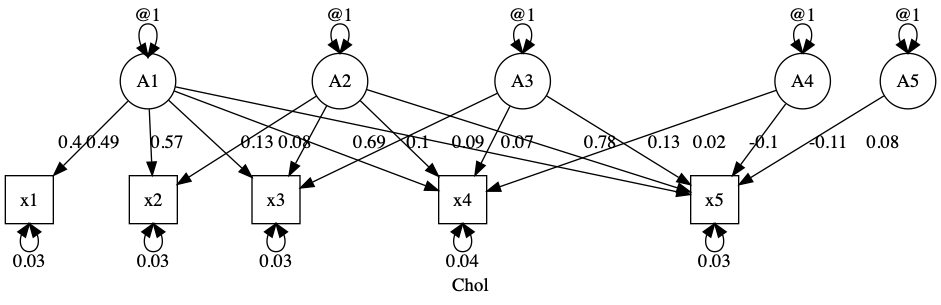# umx!

## Cholesky paths with umxPath

This page will introduce writing Cholesky structures using `umxPath` to facilitate twin modeling with `umxRAM`.

It is not finished, and for now (and perhaps for sometime), I’d recommend you read this page instead on the awesome umxACE function

``````# ====================
# = Cholesky example =
# ====================
latents   = paste0("A", 1:5)
manifests = names(demoOneFactor)
myData    = mxData(cov(demoOneFactor), type = "cov", numObs = 500)
m1 = umxRAM("Chol", data = myData,
umxPath(Cholesky = latents, to = manifests),
umxPath(var = manifests),
umxPath(var = latents, fixedAt = 1.0)
)
``````

We can see that this fits “perfectly” - it’s a saturated solution, with some caveats (see `umx::umxACEv` to learn more about these):

``````umxSummary(m1)

``````### What about a 1-factor solution?

A 1 factor Cholesky is just a general factor, but let’s use it as an example:

``````manifests = names(demoOneFactor)
m2 = umxRAM("Chol", data = demoOneFactor, type = "cov",
umxPath(Cholesky = "A1", to = manifests),
umxPath(var = manifests),
umxPath(var = "A1", fixedAt = 1)
)
plot(m2)

``````

We could also drop the paths from m1:

``````m3 = umxModify(m1, regex = "^A[2-5]")

``````

tip: use `parameters(m1, pattern = "^A[2-5]")` to see what paths this expression matches and drops.

Is a one-factor model significantly worse than the extravagant saturated five Cholesky-factor solution?

``````umxCompare(m1, m2)
``````
Model EP Δ -2LL Δ df p AIC Δ AIC Compare with Model
Chol 20       9.994993 0
Chol 10 7.3987999 10 0.687 -2.606207 -12.6012001 Chol

No: fit is not-significantly worse.

## A multi-group twin model

Twin models have models for each class of twins – MZ DZ, perhaps differentiated by sex – and these differ in the genetic paths they set.

umx includes built-in twin models, including `umxACE`. Here, we’ll build a twin model completely by hand in `umx` from scratch.

NOTE: `umx` now includes `umxTwinMaker` which makes specifying novel multivariate twin models MUCH more straight-foward.

First let’s build an ace model from scratch:

``````data(twinData)
tmpTwin = twinData
names(tmpTwin)
# "fam", "age", "zyg", "part", "wt1", "wt2", "ht1", "ht2", "htwt1", "htwt2", "bmi1", "bmi2"

# Set zygosity to a factor
labList = c("MZFF", "MZMM", "DZFF", "DZMM", "DZOS")
tmpTwin\$zyg = factor(tmpTwin\$zyg, levels = 1:5, labels = labList)

# Pick the variables
selDVs = c("bmi1", "bmi2") # nb: Can also give base name, (i.e., "bmi") AND set suffix.

# the function will then make the varnames for each twin using this:
# for example. "VarSuffix1" "VarSuffix2"
mzData = tmpTwin[tmpTwin\$zyg %in% "MZFF", selDVs]
dzData = tmpTwin[tmpTwin\$zyg %in% "DZFF", selDVs]

latentA = paste0(c("A1"), "_T", 1:2)
latentC = paste0(c("C1"), "_T", 1:2)
latentE = paste0(c("E1"), "_T", 1:2)
latents = c(latentA, latentC, latentE)

mz = umxRAM("mz", data = mxData(mzData, type = "raw"),
umxPath(v1m0 = latents),
umxPath(v.m. = selDVs),
# twin 1
umxPath(Cholesky = "A1_T1", to = "bmi1"),
umxPath(Cholesky = "C1_T1", to = "bmi1"),
umxPath(Cholesky = "E1_T1", to = "bmi1"),
# twin 2
umxPath(Cholesky = "A1_T2", to = "bmi2"),
umxPath(Cholesky = "C1_T2", to = "bmi2"),
umxPath(Cholesky = "E1_T2", to = "bmi2"),
umxPath("A1_T1", with = "A1_T2", fixedAt = 1),
umxPath("C1_T1", with = "C1_T2", fixedAt = 1),
umxPath("E1_T1", with = "E1_T2", fixedAt = 0)
)
dz = mxModel(mz, name= "dz",
mxData(dzData, type = "raw"),
umxPath("A1_T1", with = "A1_T2", fixedAt = .5)
)

m1 = mxModel(mz, dz, mxFitFunctionMultigroup(c("mz", "dz")))
parameters(mz)
plot(dz, fixed= TRUE)
``````

## umxTwinMaker

Now let’s do that in `umxTwinMaker`. Here we only have to draw the model for one person.

First let’s assemble some data

``````data(twinData)
tmp = umx_make_twin_data_nice(data=twinData, sep="", zygosity="zygosity", numbering=1:2)
tmp = umx_scale_wide_twin_data(varsToScale= c("wt", "ht"), sep= "_T", data= tmp)
mzData = subset(tmp, zygosity %in%  c("MZFF", "MZMM"))
dzData = subset(tmp, zygosity %in%  c("DZFF", "DZMM"))
``````

Now, I’ll make a bivariate ACE twin model.

### 1. Define paths for one person:

``````latents = paste0(rep(c("a", "c", "e"), each = 2), 1:2)
paths = c(
umxPath(v1m0 = latents),
umxPath(mean = c("wt", "ht")),
umxPath(fromEach = c("a1", 'c1', "e1"), to = c("ht", "wt")),
umxPath(c("a2", 'c2', "e2"), to = "wt")
)
``````

### 2. Make a twin model based on the paths for one person

``````m1 = umxTwinMaker("myACE", paths, mzData = mzData, dzData= dzData)
plot(m1, std= TRUE, means= FALSE)

``````
``````m2 = umxACE(selDVs= c("wt", "ht"), mzData = mzData, dzData=dzData, sep="_T")